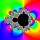# Geometric progression 2

There is geometric sequence with a1=5.7 and quotient q=-2.5. Calculate a17.

Result

a17 =  13271346.69

#### Solution:Leave us a comment of example and its solution (i.e. if it is still somewhat unclear...):Be the first to comment!## Next similar examples:

1. Tenth memberCalculate the tenth member of geometric sequence when given: a1=1/2 and q=2
2. Geometric sequence 4It is given geometric sequence a3 = 7 and a12 = 3. Calculate s23 (= sum of the first 23 members of the sequence).
3. Geometric sequence 5About members of geometric sequence we know: ? ? Calculate a1 (first member) and q (common ratio or q-coefficient)
4. Six termsFind the first six terms of the sequence a1 = -3, an = 2 * an-1
5. SequenceFind the common ratio of the sequence -3, -1.5, -0.75, -0.375, -0.1875. Ratio write as decimal number rounded to tenth.
6. Five membersWrite first 5 members geometric sequence and determine whether it is increasing or decreasing: a1 = 3 q = -2
7. GP membersThe geometric sequence has 10 members. The last two members are 2 and -1. Which member is -1/16?
8. Sequence - 5 membersWrite first five members of the sequence ?
9. PopulationWhat is the population of the city with 3% annual growth, if in 10 years the city will have 60,000 residents?
10. Geometric progression 48,4√2,4,2√2
11. GP - 8 itemsDetermine the first eight members of a geometric progression if a9=512, q=2
12. A perineumA perineum string is 10% shorter than its original string. The first string is 24, what is the 9th string or term?
13. Geometric progressionFill 4 numbers between 4 and -12500 to form geometric progression.
14. AS sequenceIn an arithmetic sequence is given the difference d = -3 and a71 = 455. a) Determine the value of a62 b) Determine the sum of 71 members.
15. CalculationHow much is sum of square root of six and the square root of 225?
16. Theorem proveWe want to prove the sentence: If the natural number n is divisible by six, then n is divisible by three. From what assumption we started?
17. TrinityHow many different triads can be selected from the group 43 students?